AP State Syllabus AP Board 7th Class Maths Solutions Chapter 4 Lines and Angles Ex 7 Textbook Questions and Answers.

## AP State Syllabus 7th Class Maths Solutions 4th Lesson Lines and Angles Exercise 7Question 1.
Fill up the blanks
(i) The line which intersects two or more lines at distinct points is called __________
(ii) If the pair of alternate interior angles are equal then the lines are ______________
(iii) The sum of interior angles on the same side of the transversal are supplementary then the lines are ____________
(iv) If two lines intersect each other then the number of common points they have
Solution:
i) The line which intersects two or more lines at distinct points is called transversal.
ii) If the pairs of alternate interior angles are equal then the lines are parallel.
ii) The sum of interior angles on the same side of the transversal are supplementary then the lines are parallel.
iv) If two lines intersect each other then the number of common points they have Only one.Question 2.
In the adjacent figure, the lines ‘P and ‘m’ are parallel and ‘n’ is a transversal. Fill in the blanks for all the situations given below.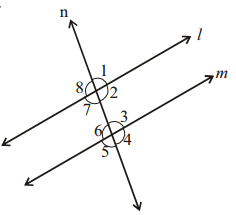(i) If ∠1 = 80° then∠2 =
(ii) If ∠3 = 45° then ∠7 =
(iii) If ∠2 = 90° then ∠8 =
(iv) If ∠4 = 100° then ∠8 =
Solution:
(i) If ∠1 = 80° then∠2 = 100
(ii) If ∠3 = 45° then ∠7 = 45
(iii) If ∠2 = 90° then ∠8 = 90
(iv) If ∠4 = 100° then ∠8 = 100

Question 3.
Find the measures of x,y and z in the figure, where l || BC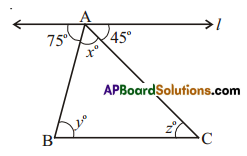Solution:
∠y = 75° (alt. tnt. angles)
∠z = 45° (ait. tnt. angles)
∠x + ∠y + ∠z = 180° (∵ int. angles of a triangle = 1800)
75° + 45° + ∠x = 180°
∠x = 180°- 120°
= 60°Question 4.
ABCD is a quadrilateral in which AB || DC and AD || BC. Find ∠b, ∠c and ∠d.
Solution:
∠b + ∠50° = 180° (int. angles on the same side of AB)
∠b = 180° – 50° = 130°
Similarly ∠b + ∠c = ∠c + ∠d = ∠d + ∠50 = 180°
∠130° + ∠c = 180°
∠c = 180° – 130° = 50°
Also ∠c + ∠d = 50° +∠d = 180°
∠d = 180° – 50° = 130°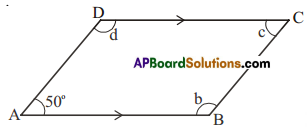Question 5.
In a given figure, ‘l’ and m’ are intersected by a transversal ‘n’. Is 1 || m?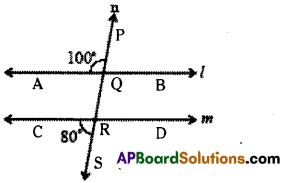Solution:
∠AQP and ∠CRS are exterior angles on the same side of the transversal n and their sum
100° + 80° = 180°
Hence l//m.Question 6.
Find ∠a, ∠b, ∠c, ∠d and ∠e in the figure? Give reasons.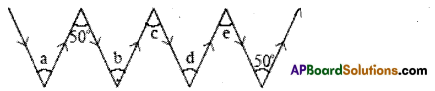Note: Two anow marks pointing in the same direction represent parallel lines.
Solution:
∠a = ∠50° (∵ alt. int, angle between two parallel lines)
∠b = 50° (∵ alt. int, angle between two parallel lines)
∠b = ∠c = 50° (∵ alt. int. angles between two parallel lines)
∠c = ∠d = 50° ( ∵ alt. int angles between two parallel lines)
∠e = ∠d = 50°(∵ alt. int. angles between two parallel lines)